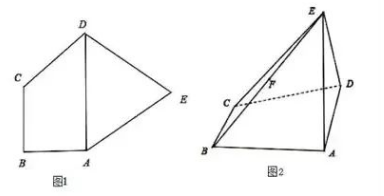$\triangle A D E$ 是等边三角形. 现将 $\triangle A D E$ 沿 $A D$ 折 起, 连接 $E B, E C$ 得四棱雉 $E-A B C D$ (如 图 2）, 且 $E C=2 \sqrt{2}$.
(1) 求证: 平面 $E A D \perp$ 平面 $A B C D$;
(2) 若 $F$ 是棱 $E B$ 的中点, 求 $C F$ 与平面 $A B C D$ 所成角的正切值.【答案】 (1) 取 $A D$ 中点 $O$, 连接 $O C, O E$, 易得 $O E \perp A D, O C \perp A D$.

\begin{aligned} & \because O C^2+O E^2=C E^2 \\ & \therefore O E \perp O C, \\ & \text { 又 } O E \perp A D, \\ & O C \cap A D=O \ldots \ldots \ldots . \end{aligned}

(2) 由 $F$ 是棱 $E B$ 的中点, 令 $\mathrm{OB}$ 的中点为 $\mathrm{K}$, 连接 $\mathrm{FK}$.